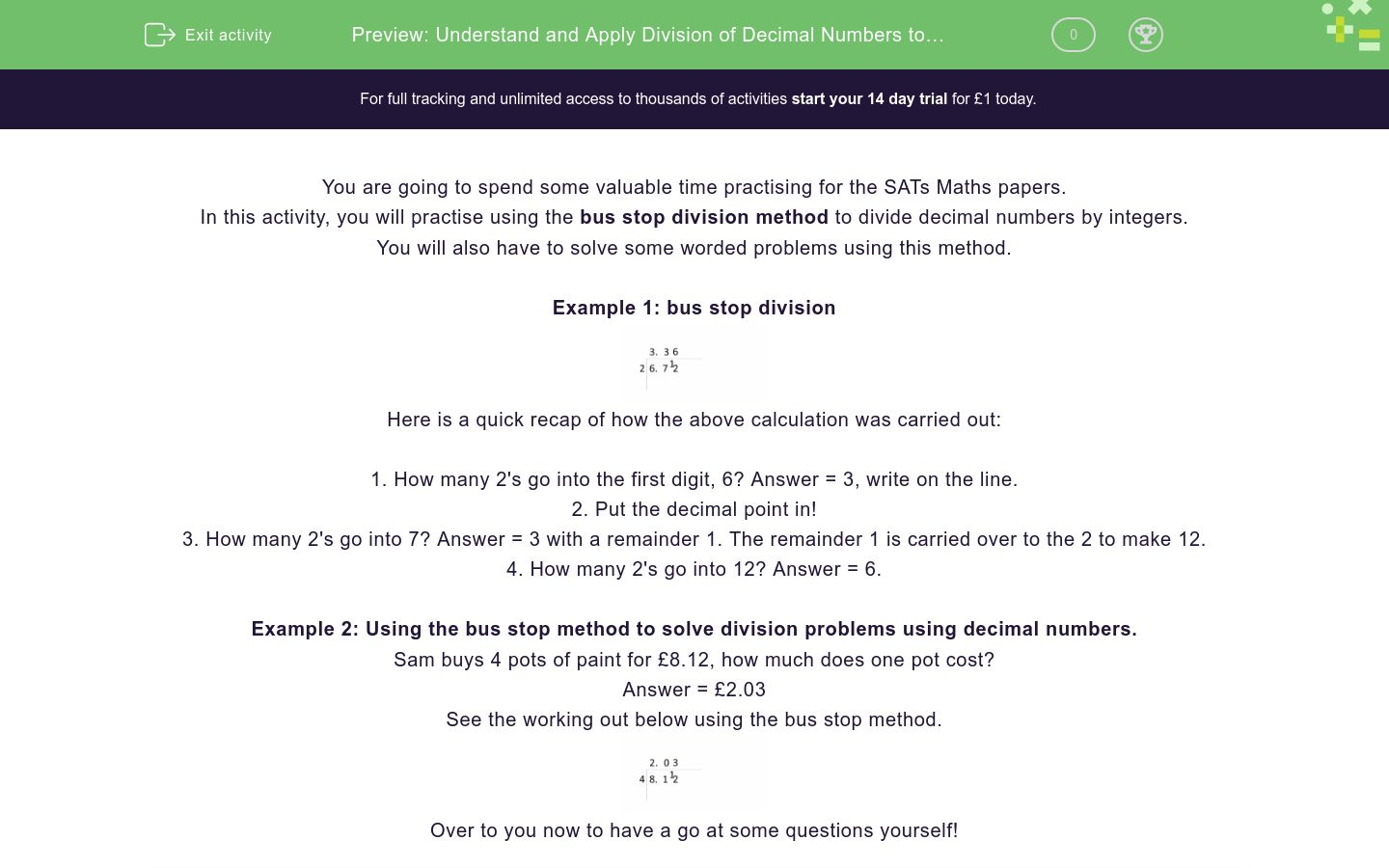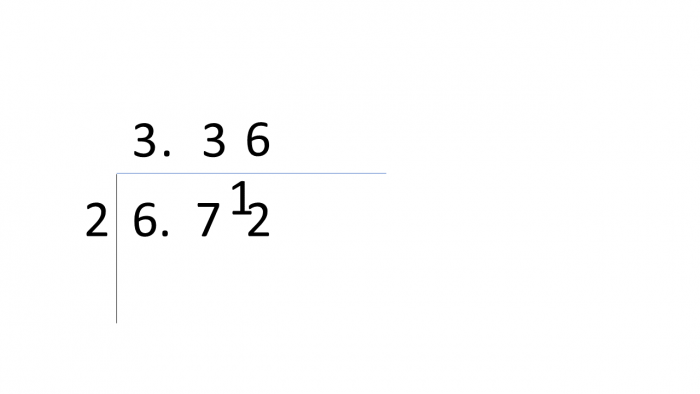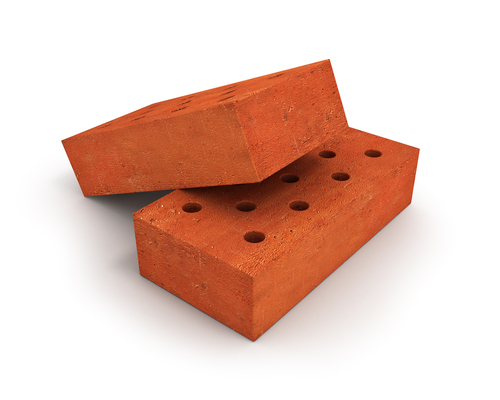# Understand and Apply Division of Decimal Numbers to Solve Problems

In this worksheet, students will be asked to divide decimal numbers by an integer. Students will also use this skill to solve a range of worded problems. This is a key skill to cement in preparation for SATs Maths tests.Key stage:  KS 2

Curriculum topic:   Exam-style Questions: SATs Maths

Curriculum subtopic:   Exam-Style Questions: Accurate Decimal Calculations

Difficulty level:### QUESTION 1 of 10

You are going to spend some valuable time practising for the SATs Maths papers.

In this activity, you will practise using the bus stop division method to divide decimal numbers by integers.

You will also have to solve some worded problems using this method.

Example 1: bus stop divisionHere is a quick recap of how the above calculation was carried out:

1. How many 2's go into the first digit, 6? Answer = 3, write on the line.

2. Put the decimal point in!

3. How many 2's go into 7? Answer = 3 with a remainder 1. The remainder 1 is carried over to the 2 to make 12.

4. How many 2's go into 12? Answer = 6.

Example 2: Using the bus stop method to solve division problems using decimal numbers.

Sam buys 4 pots of paint for £8.12, how much does one pot cost?

See the working out below using the bus stop method.Over to you now to have a go at some questions yourself!

Can you choose the correct answer for this calculation?

6.69 ÷ 3 = ?

2.03

3.23

2.23

3.03

Divide 9.12 by 4.

Match each question with its answer.

Make sure you do some working out, using the bus stop method for each one.

## Column B

12.4 ÷ 2 =
0.71
6.84 ÷ 4 =
6.2
4.26 ÷ 6 =
1.71

Solve 9.55 ÷ 5 = ?

Tim says, "9.88 ÷ 4 = 2.48".

Three bars of chocolate cost £5.85.

How much does one chocolate bar cost?Can you match the answer with the correct calculation?

## Column B

2.3 ÷ 5 =
0.46
15.6 ÷ 3 =
5.2
8.28 ÷ 4 =
2.07

Three children decide to join together to buy a Christmas present for their parents.

The cost of the present is £36.54

How much does each child pay?## Column B

2.3 ÷ 5 =
0.46
15.6 ÷ 3 =
5.2
8.28 ÷ 4 =
2.07

A builder spends £202.44 on bricks.

He uses these bricks to build 4 walls.

How much does it cost to build 1 wall?## Column B

2.3 ÷ 5 =
0.46
15.6 ÷ 3 =
5.2
8.28 ÷ 4 =
2.07

A group of 8 friends spend £60.48 on food and prizes for their party.

How much does each child pay towards the party?## Column B

2.3 ÷ 5 =
0.46
15.6 ÷ 3 =
5.2
8.28 ÷ 4 =
2.07
• Question 1

Can you choose the correct answer for this calculation?

6.69 ÷ 3 = ?

2.23
EDDIE SAYS
How did you get on with this first question? The answer is 2.23. The most accurate way to divide a decimal number is by using the bus stop method. You've got going now, let's push on.
• Question 2

Divide 9.12 by 4.

2.28
EDDIE SAYS
Nice job if you worked out the answer of 2.28. Hopefully, you are getting the hang of bus stop division, it really is an efficient and accurate way to divide!
• Question 3

Match each question with its answer.

Make sure you do some working out, using the bus stop method for each one.

## Column B

12.4 ÷ 2 =
6.2
6.84 ÷ 4 =
1.71
4.26 ÷ 6 =
0.71
EDDIE SAYS
Is this becoming less daunting? There were three questions to tackle there but remember... practice really does make perfect! Hopefully, by now you've noticed a good knowledge of times tables really helps when using the bus stop method, making it even quicker to answer a question!
• Question 4

Solve 9.55 ÷ 5 = ?

1.91
EDDIE SAYS
Did you reach the answer of 1.91? Don't forget to include the decimal point when dividing a decimal number or your answer will be incorrect.
• Question 5

Tim says, "9.88 ÷ 4 = 2.48".

EDDIE SAYS
There are 2 marks available for this question. The first mark is for recognising that Tim is incorrect. The second mark is for proving why. The student can use the bus stop method to demonstrate that 9.88 ÷ 4 = 2.47.
• Question 6

Three bars of chocolate cost £5.85.

How much does one chocolate bar cost?EDDIE SAYS
To crack this one, you had to divide £5.85 by 3. Using the bus stop method would most certainly be the quickest and most accurate method here. You are more than halfway through this activity- keep making progress.
• Question 7

Can you match the answer with the correct calculation?

## Column B

2.3 ÷ 5 =
0.46
15.6 ÷ 3 =
5.2
8.28 ÷ 4 =
2.07
EDDIE SAYS
Remember to take your time and use the bus stop method to divide carefully. Always leave the decimal point where it is and don't forget to include it in your answer!
• Question 8

Three children decide to join together to buy a Christmas present for their parents.

The cost of the present is £36.54

How much does each child pay?EDDIE SAYS
Great job if you wrote £12.18. To crack this one, you had to calculate £36.54 ÷ 3, this equals £12.18. Keep going, only two questions left!
• Question 9

A builder spends £202.44 on bricks.

He uses these bricks to build 4 walls.

How much does it cost to build 1 wall?EDDIE SAYS
Did you read the question carefully and calculate the sum £202.44 ÷ 4 = £50.61?
• Question 10

A group of 8 friends spend £60.48 on food and prizes for their party.

How much does each child pay towards the party?EDDIE SAYS
Don't be afraid to give it a go! This time the calculation needed was: £60.48 ÷ 8 = £7.56 I'm sure you have really got the hang of how to divide decimal numbers now! Great work, well done for completing another activity! You're now more prepared for your SATs Maths tests.